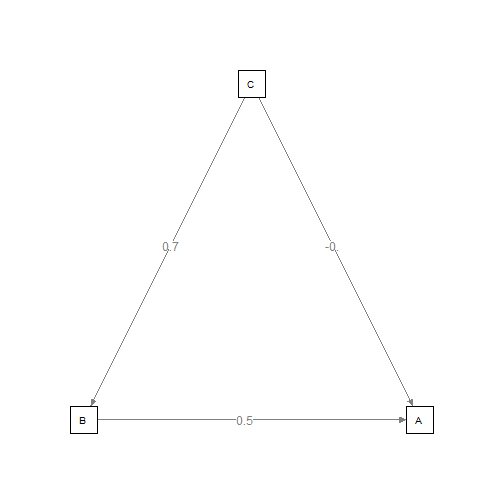semPlot-tricks {semPlot} R Documentation

Tricks that can be used in semPlot.

Description

Use a list contaning several SEM objects (from any source) to plot them as the same model. Also, the '+' operator can be used to combine two models, including in calls in `semPaths` and `semPlotModel`. See examples.

Usage

```## S3 method for class 'semPlotModel'
x + y
## S3 method for class 'list'
semPlotModel(object)
```

Arguments

 `x` A `"semPlotModel"` object `y` A `"semPlotModel"` object `object` An object contaning the result of a SEM or GLM analysis, or a string contaning the file path to the output file of a sEM program.

Author(s)

Sacha Epskamp <mail@sachaepskamp.com>

`semPlotModel` `semPaths` `semCors`

Examples

``````
# A silly dataset:
A <- rnorm(100)
B <- A + rnorm(100)
C <- B + rnorm(100)
DF <- data.frame(A,B,C)

# Two regressions:
res1 <- lm(B ~ C, data = DF)
res2 <- lm(A ~ B + C, data = DF)

# Plot both in the same path diagram in two ways:
semPaths(res1 + res2, "model", "est", intercepts=FALSE)
````````````semPaths(list(res1,res2), "model", "est", intercepts=FALSE)

``````

[Package semPlot version 0.3 Index]